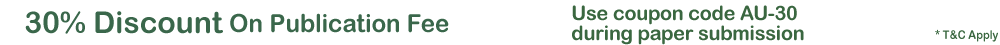Quality Publication | Affordable Price | Happy AuthorsDeterministic Mathematical Model Of Zika Virus
A deterministic mathematical model describing the dynamics of Zika virus using a set of ordinary differential equation was developed. The basic reproductive number of the model which is an important threshold for disease control was obtained. The model has disease free equilibrium which is stable whenever . Analysis shows that the endemic equilibrium exists and the conditions for its stability or otherwise were given. Further, a numerical value for was computed and sensitivity analysis of its parameters shows that the progression rate from Exposed to Infected mosquito is the most sensitive parameter to